# 混沌操作法策略源码解析

Author: Hukybo, Created: 2020-01-11 16:57:47, Updated: 2020-01-16 14:44:49

#### 三. 混沌操作法架构

• 鳄鱼线(Alligator)
• 碎形(The Fractal)
• 动量(The Momentum)
• 加速(Acceleration)
• 均衡线(The Balance Line)

#### 鳄鱼线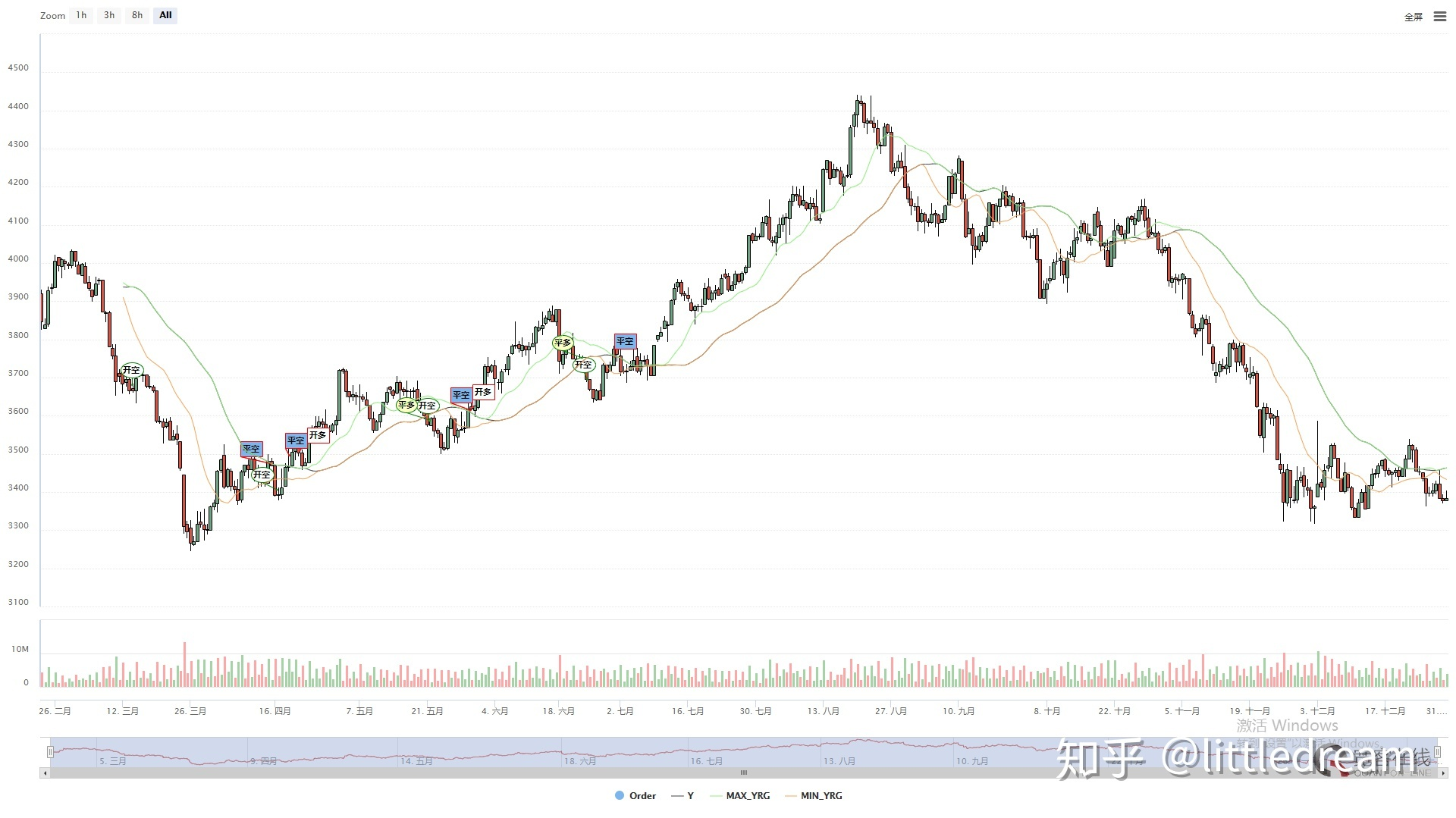``````// 参数
N1:=11;
N2:=21;

// 定义价格中线
N3:=N1+N2;
N4:=N2+N3;
HL:=(H+L)/2;

// 鳄鱼线
Y^^SMA(REF(HL,N3),N4,1);
R:=SMA(REF(HL,N2),N3,1);
G:=SMA(REF(HL,N1),N2,1);
``````

#### 四. 分形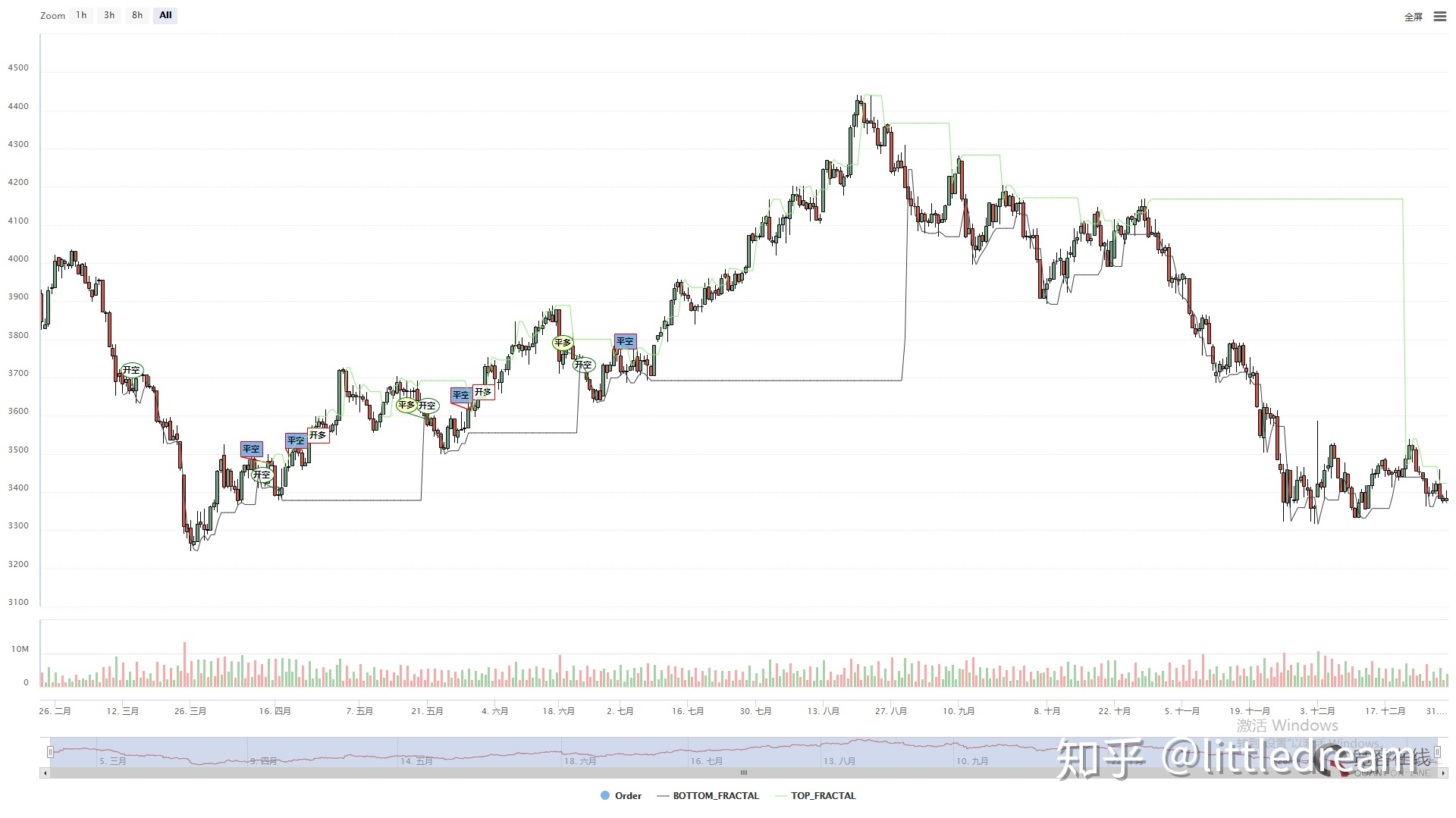``````// 分形
TOP_N:=BARSLAST(REF(H,2)=HHV(H,5))+2;
BOTTOM_N:=BARSLAST(REF(L,2)=LLV(L,5))+2;

TOP:=REF(H,TOP_N);
BOTTOM:=REF(L,BOTTOM_N);

MAX_YRG^^MAX(MAX(Y,R),G);
MIN_YRG^^MIN(MIN(Y,R),G);

TOP_FRACTAL^^VALUEWHEN(H>=MAX_YRG,TOP);
BOTTOM_FRACTAL^^VALUEWHEN(L<=MIN_YRG,BOTTOM);
``````

#### 五. 策略逻辑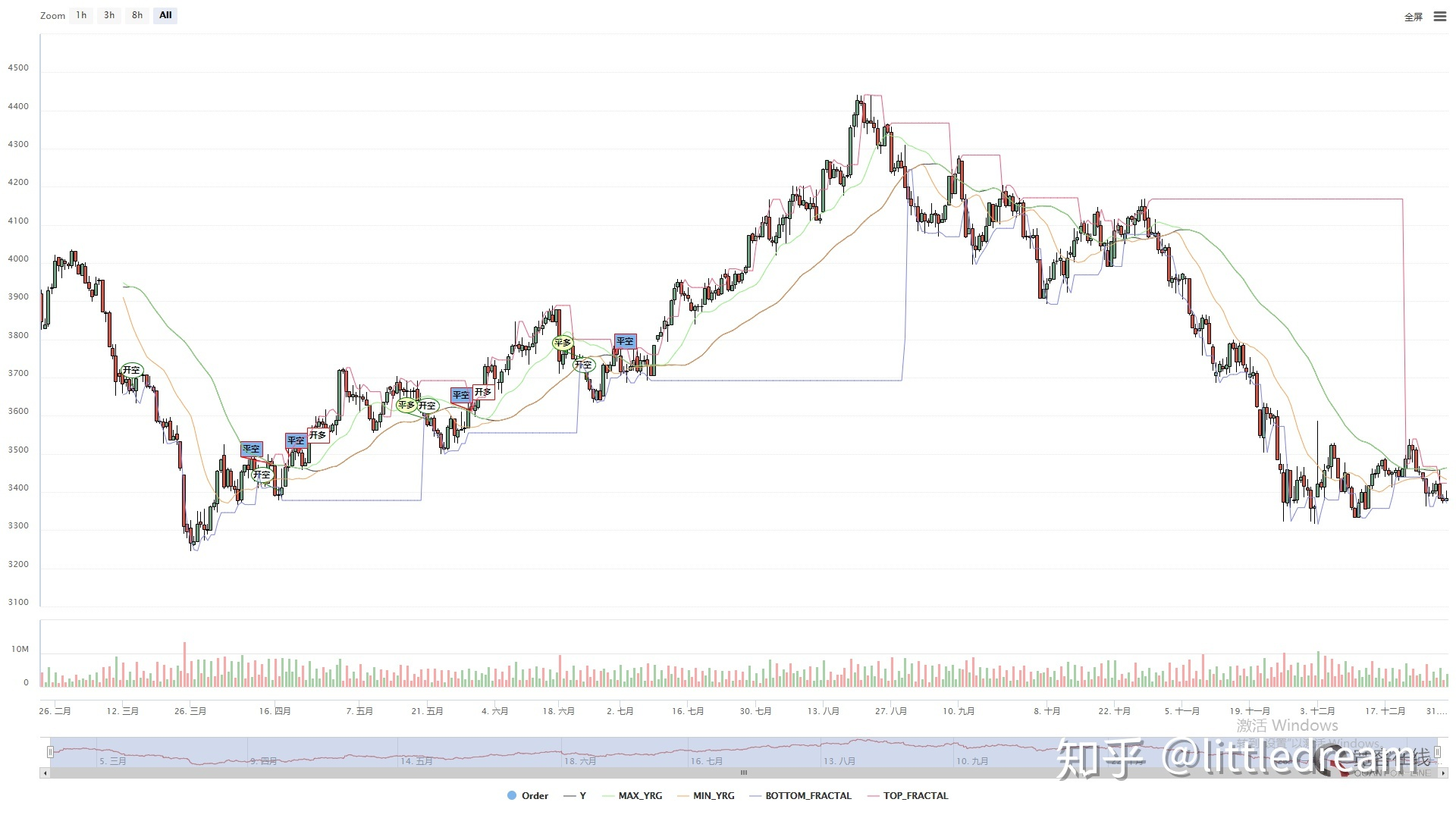``````// 如果当前无多单，并且收盘价升破上分形，并且上分形在鳄鱼线上方时，多头开仓
BKVOL=0 AND C>=TOP_FRACTAL AND TOP_FRACTAL>MAX_YRG,BPK(1);
// 如果当前无空单，并且收盘价跌破下分形，并且下分形在鳄鱼线下方时，空头开仓
SKVOL=0 AND C<=BOTTOM_FRACTAL AND BOTTOM_FRACTAL<MIN_YRG,SPK(1);

// 如果收盘价跌破鳄鱼的下巴时，多头平仓
C<Y,SP(BKVOL);
// 如果收盘价升破鳄鱼的下巴时，空头平仓
C>Y,BP(SKVOL);
``````
• 多头开仓：如果当前无多单，并且收盘价升破上分形，并且上分形在鳄鱼线上方。
• 空头开仓：如果当前无空单，并且收盘价跌破下分形，并且下分形在鳄鱼线下方。
• 多头平仓：如果收盘价跌破鳄鱼下巴。
• 空头平仓：如果收盘价升破鳄鱼下巴。

#### 六. 策略源码

``````(*backtest
start: 2010-01-01 09:00:00
end: 2019-01-01 15:00:00
period: 1d
exchanges: [{"eid":"Futures_CTP","currency":"FUTURES","minfee":10}]
args: [["N1",11],["N2",21],["ContractType","rb000",126961]]
*)

N3:=N1+N2;
N4:=N2+N3;

HL:=(H+L)/2;

// 鳄鱼线
Y^^SMA(REF(HL,N3),N4,1);
R:=SMA(REF(HL,N2),N3,1);
G:=SMA(REF(HL,N1),N2,1);

// 分形
TOP_N:=BARSLAST(REF(H,2)=HHV(H,5))+2;
BOTTOM_N:=BARSLAST(REF(L,2)=LLV(L,5))+2;

TOP:=REF(H,TOP_N);
BOTTOM:=REF(L,BOTTOM_N);

MAX_YRG^^MAX(MAX(Y,R),G);
MIN_YRG^^MIN(MIN(Y,R),G);

TOP_FRACTAL^^VALUEWHEN(H>=MAX_YRG,TOP);
BOTTOM_FRACTAL^^VALUEWHEN(L<=MIN_YRG,BOTTOM);

// 如果当前无多单，并且收盘价升破上分形，并且上分形在鳄鱼线上方时，多头开仓
BKVOL=0 AND C>=TOP_FRACTAL AND TOP_FRACTAL>MAX_YRG,BPK(1);
// 如果当前无空单，并且收盘价跌破下分形，并且下分形在鳄鱼线下方时，空头开仓
SKVOL=0 AND C<=BOTTOM_FRACTAL AND BOTTOM_FRACTAL<MIN_YRG,SPK(1);

// 如果收盘价跌破鳄鱼的下巴时，多头平仓
C<Y,SP(BKVOL);
// 如果收盘价升破鳄鱼的下巴时，空头平仓
C>Y,BP(SKVOL);
``````

#### 七. 回测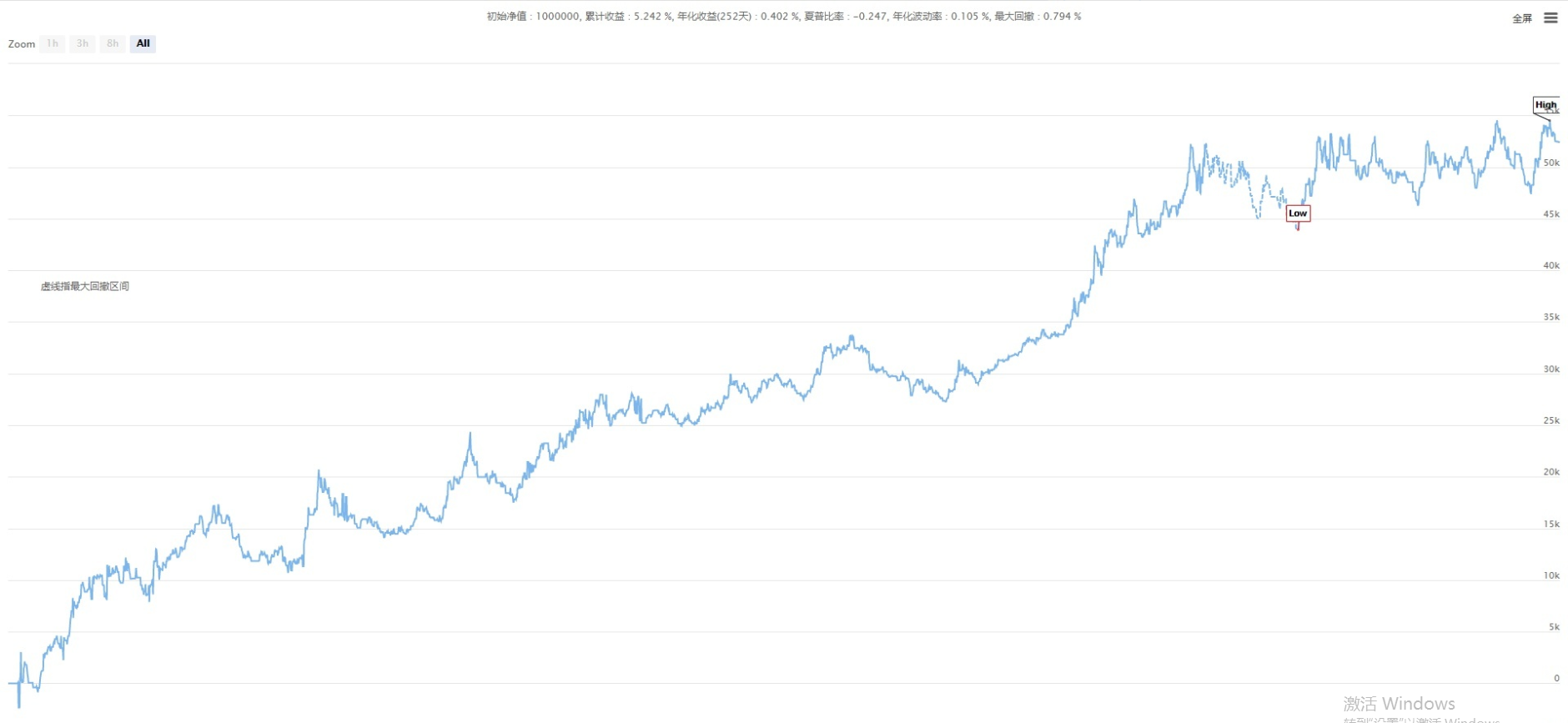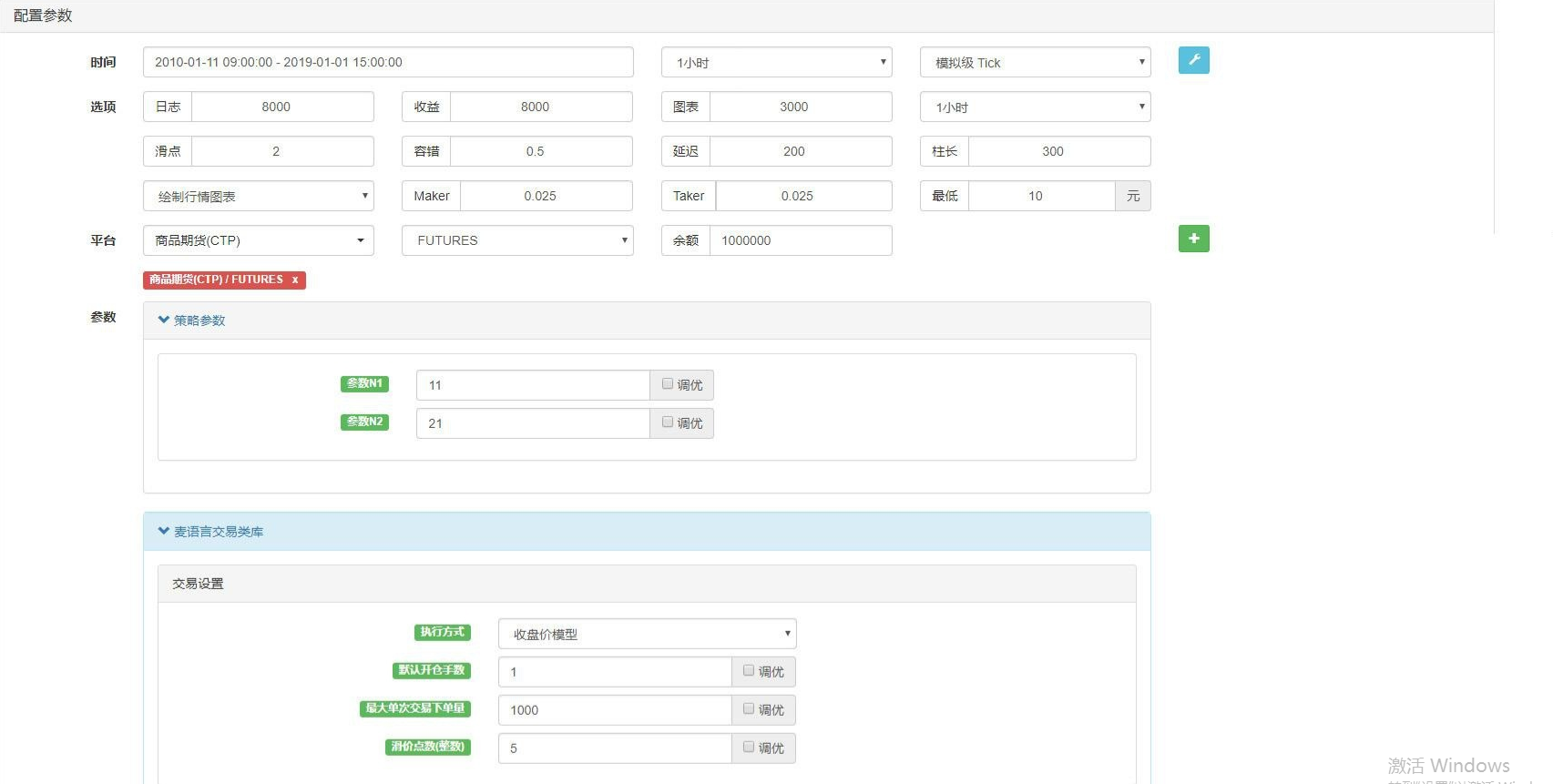Related

More